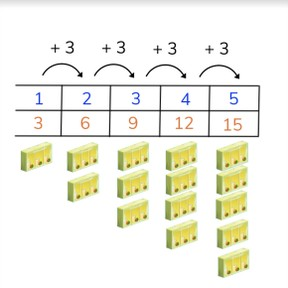Ratio tables 1 - 5

# Ratio tables 1 - 5

Students learn to create and solve ratio tables with groups of 1-5

No account needed.8,000 schools use Gynzy92,000 teachers use Gynzy1,600,000 students use Gynzy

## General

Students will be introduced to ratio tables and learn how to make a ratio table with simple numbers 1-5 and complete simple ratio tables.

## Standards

CCSS.Math.Content.6.RPA.3.A

## Learning objective

Students will be able to make a ratio table with simple numbers 1-5.

## Introduction

Start the lesson by showing the students the image of the animals in the barn. Have students count the pigs, and have them stand when they've counted all of the pigs. Did they count carefully enough? Then have students circle pairs of chickens in the next image. How many pairs do they have? How many chickens are there? You ca discuss different forms of counting here if you wish.

## Instruction

Explain the importance of ratio tables to the students. Explain to students what a ratio table is, namely a table that shows how many groups you have, and how many of the objects are in each group. Show this using the example with the juice boxes. Walk through each part of the ratio table. Then show students that it is possible to skip a step in the ratio table, they must then solve the missing step in their heads. As a class, practice with the next two ratio tables and see if students can come up with the missing numbers in the ratio table. Then show students that you can also have a vertical ratio table. Walk through the vertical ratio table as a class. Then ask students individually or in pairs if they can complete the second blank vertical ratio table.

Check that students are able to make a ratio table with numbers 1-5 by asking the following questions:
- What is does the top row of a (horizontal) ratio table tell us?
- What does the bottom row of a (horizontal) ratio table tell us?

## Quiz

Students are given ten questions in which they must complete the ratio tables. Some of the questions ask students to take information from a picture, other times from text.

## Closing

Remind students why ratio tables are useful, you can for example determine how many packages of juice boxes you need to buy. Close the lesson by having students create ratio tables using the body. An example is given with legs. Ask students to form pairs and to create a few more ratio tables based on their bodies. (fingers, toes, eyes, ears, noses, etc).

## Teaching tips

Students who have difficulty with this learning goal can be supported by recreating the ratio table using math manipulatives like blocks or marbles to physically represent the ratios.

## Instruction materials

Math manipulatives like blocks or marbles.

### The online teaching platform for interactive whiteboards and displays in schools

• Save time building lessons

• Manage the classroom more efficiently

• Increase student engagement